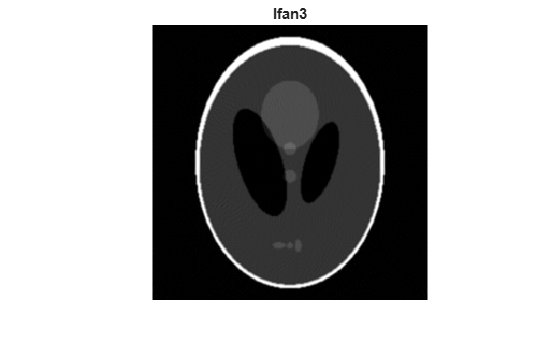## Fan-Beam Projection

Note

For information about creating projection data from line integrals along parallel paths, see Radon Transform. To convert fan-beam projection data to parallel-beam projection data, use the `fan2para` function.

The `fanbeam` function computes projections of an image matrix along specified directions. A projection of a two-dimensional function f(x,y) is a set of line integrals. The `fanbeam` function computes the line integrals along paths that radiate from a single source, forming a fan shape. To represent an image, the `fanbeam` function takes multiple projections of the image from different angles by rotating the source around the center of the image. The following figure shows a single fan-beam projection at a specified rotation angle.

Fan-Beam Projection at Rotation Angle ThetaWhen you compute fan-beam projection data using the `fanbeam` function, you specify as arguments an image and the distance between the vertex of the fan-beam projections and the center of rotation (the center pixel in the image). The `fanbeam` function determines the number of beams, based on the size of the image and the values of specified name-value arguments.

The `FanSensorGeometry` name-value argument specifies how sensors are aligned: `"arc"` or `"line"`.

Fan Sensor GeometryDescription
`"arc"``fanbeam` positions the sensors along an arc, spacing the sensors at 1 degree intervals. Use the `FanSensorSpacing` parameter to control the distance between sensors by specifying the angle between each beam. This is the default fan sensor geometry.
`"line"``fanbeam` positions sensors along a straight line, rather than an arc. Use the `FanSensorSpacing` parameter to specify the distance between the sensors, in pixels, along the x´ axis.

The `FanRotationIncrement` parameter specifies the rotation angle increment. By default, `fanbeam` takes projections at different angles by rotating the source around the center pixel at 1 degree intervals.

The following figures illustrate both these geometries. The first figure illustrates geometry used by the `fanbeam` function when `FanSensorGeometry` is set to `"arc"` (the default). Note how you specify the distance between sensors by specifying the angular spacing of the beams.

Fan-Beam Projection with Arc Geometry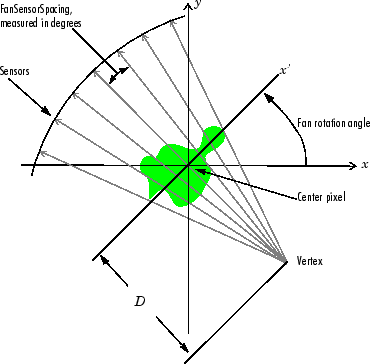The following figure illustrates the geometry used by the `fanbeam` function when `FanSensorGeometry` is set to `"line"`. In this figure, note how you specify the position of the sensors by specifying the distance between them in pixels along the x´ axis.

Fan-Beam Projection with Line Geometry### Image Reconstruction from Fan-Beam Projection Data

To reconstruct an image from fan-beam projection data, use the `ifanbeam` function. With this function, you specify as arguments the projection data and the distance between the vertex of the fan-beam projections and the center of rotation when the projection data was created. For example, this code recreates the image `I` from the projection data `P` and distance `D`.

`I = ifanbeam(P,D);`

By default, the `ifanbeam` function assumes that the fan-beam projection data was created using the arc fan sensor geometry, with beams spaced at 1 degree angles and projections taken at 1 degree increments over a full 360 degree range. As with the `fanbeam` function, you can use `ifanbeam` name-value arguments to specify other values for these characteristics of the projection data. Use the same values for these name-value arguments that were used when the projection data was created. For more information about these parameters, see `ifanbeam`.

The `ifanbeam` function converts the fan-beam projection data to parallel-beam projection data with the `fan2para` function, and then calls the `iradon` function to perform the image reconstruction. For this reason, the `ifanbeam` function supports certain `iradon` parameters, which it passes to the `iradon` function. See The Inverse Radon Transformation for more information about the `iradon` function.

### Reconstruct Image using Inverse Fanbeam Projection

This example shows how to use `fanbeam` and `ifanbeam` to form projections from a sample image and then reconstruct the image from the projections.

Create a test image of the Shepp-Logan head phantom using the `phantom` function. The phantom image illustrates many of the qualities that are found in real-world tomographic imaging of human heads.

```P = phantom(256); imshow(P)```Compute fan-beam projection data of the test image, using the `FanSensorSpacing` name-value argument to vary the sensor spacing. The example uses the fanbeam arc geometry, so you specify the spacing between sensors by specifying the angular spacing of the beams. The first call spaces the beams at 2 degrees; the second at 1 degree; and the third at 0.25 degrees. In each call, the distance between the center of rotation and vertex of the projections is constant at 250 pixels. In addition, `fanbeam` rotates the projection around the center pixel at 1 degree increments.

```D = 250; dsensor1 = 2; F1 = fanbeam(P,D,"FanSensorSpacing",dsensor1); dsensor2 = 1; F2 = fanbeam(P,D,"FanSensorSpacing",dsensor2); dsensor3 = 0.25; [F3,sensor_pos3,rot_angles3] = fanbeam(P,D,"FanSensorSpacing",dsensor3);```

Plot the projection data `F3`. Because `fanbeam` calculates projection data at rotation angles from 0 to 360 degrees, the same patterns occur at an offset of 180 degrees. The same features are being sampled from both sides.

```figure imagesc(rot_angles3,sensor_pos3,F3) colormap(hot); colorbar xlabel("Fan Rotation Angle (degrees)") ylabel("Fan Sensor Position (degrees)")```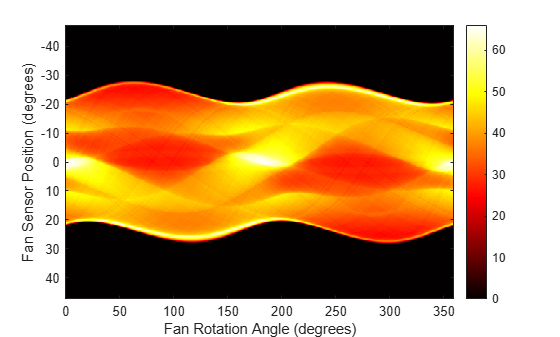Reconstruct the image from the fan-beam projection data using `ifanbeam`. In each reconstruction, match the fan sensor spacing with the spacing used when the projection data was created previously. The example uses the `OutputSize` name-value argument to constrain the output size of each reconstruction to be the same as the size of the original image `P`. In the output, note how the quality of the reconstruction gets better as the number of beams in the projection increases. The first image, `Ifan1`, was created using 2 degree spacing of the beams; the second image, `Ifan2`, was created using 1 degree spacing of the beams; the third image, `Ifan3`, was created using 0.25 spacing of the beams.

```output_size = max(size(P)); Ifan1 = ifanbeam(F1,D, ... "FanSensorSpacing",dsensor1,"OutputSize",output_size); figure, imshow(Ifan1) title("Ifan1")```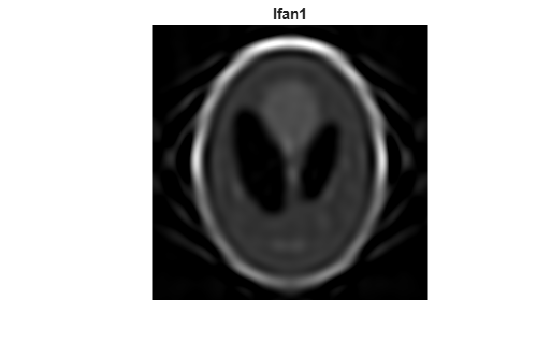```Ifan2 = ifanbeam(F2,D, ... "FanSensorSpacing",dsensor2,"OutputSize",output_size); figure, imshow(Ifan2) title("Ifan2")```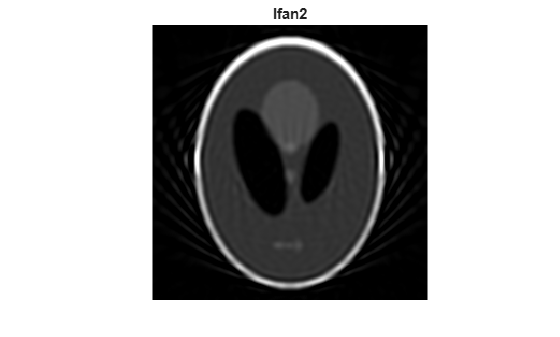```Ifan3 = ifanbeam(F3,D, ... "FanSensorSpacing",dsensor3,"OutputSize",output_size); figure, imshow(Ifan3) title("Ifan3")```